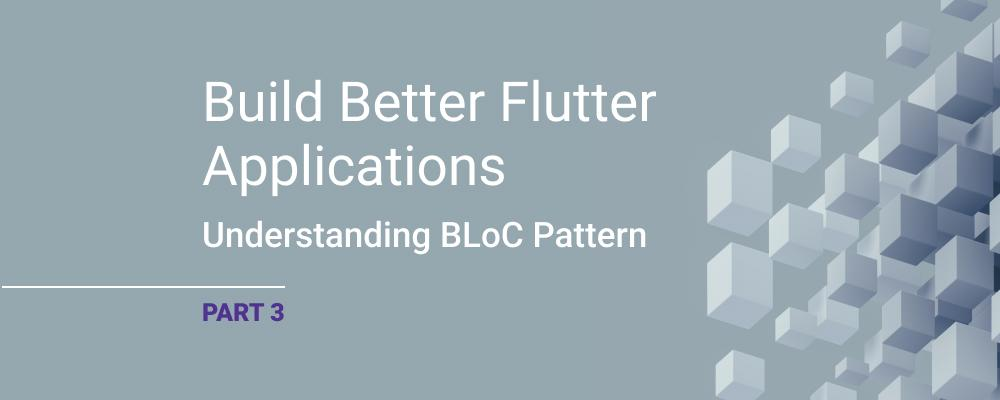# How to Conduct Unit Tests Using BLoC

In this series on BLoC for Flutter, we’ve examined differences between reactive and traditional programming methods, explored the BLoC architecture, and learned about BLoC widgets. (If you missed it, read Part 1 - Discover BLoC Pattern and Part 2 - A Deeper Look at BLoC Pattern.)

In this installment, I’ll explain how to conduct unit tests with BLoC. This is a critical step in the development process because unit tests can have many unpredictable bugs. Particularly in reactive programming constructs, the code is a bit harder to understand and therefore more error-prone. Let's get started.

## Bloc Testing

In Flutter, the bloc_test package is used to test the BLoC architecture. This package provides several helper classes for testing BLoCs. The following steps show how to test a BLoC using bloc_test. First, let’s discuss the functions used to test the Bloc architecture:

### group

The group function is used to divide tests into logical groups. This helps make your test reports more readable. You can also use functions such as setUp and tearDown that can apply to all tests in a set of tests.

### setUp

The setUp function is a function that is run before each test starts. This can be used to create common states between tests. For example, instead of creating a separate CounterBloc instance for each test, you can use the setUp function to create a single CounterBloc instance for all tests.

### tearDown

The tearDown function is a function that is run every time a test is completed. This can be used to clean up resources created after tests. For example, you can use the tearDown function to turn off the CounterBloc instance after each test.

### test

The test function is the base function for you to define and run your tests. To create a test function, you must call the test function and pass two parameters: a String value (this determines the name or description of the test) and a Function value (this is a function that contains the test's code).

### blocTest

The blocTest function is used to test BLoCs. This allows you to define a test case with input events and expected outputs that determine what states or errors the BLoC should generate. The build parameter provides a function to build the BLoC. The act parameter is a function to add an event to the BLoC. The expect parameter specifies the expected output of the BLoC and is given as a list. This allows you to check all the states that the BLoC generates in the correct order.

To use bloc_test, we need to add the bloc_test package to the pubspec.yaml file. When you are in the project directory, it will be enough to run the following command from the console:

It is also a good idea to get the Equatable package to be able to use equations:

Now we can use the bloc_test package.

Recall the CounterCubit class, which is extended from Cubit<int>, which we gave an example of before:

import 'package:flutter_bloc/flutter_bloc.dart';

class CounterCubit extends Cubit<CounterState> {
CounterCubit() : super(CounterState(0));

void increment() {
emit(CounterState(state.count + 1));
}

void decrement() {
emit(CounterState(state.count - 1));
}
}
class CounterState {
final int count;

CounterState(required this.count);
}

We need to extend our Equatable class to our CounterState class. The new CounterState class should be like the following code:

class CounterState extends Equatable{
final int count;

CounterState(required this.count);

@override
List<Object> get props => [ this.count ];
}

The second main issue is that States must return true inside the main function. If the main function does not do so, the test will fail. Here’s an example of how to use it:

void main() {
final CounterState state1 = CounterState(count:1);
final CounterState state2 = CounterState(count:1);
print(state1 == state2);
runApp(const MyApp());
}

The test code for the CounterCubit class can be as follows:

group('CounterCubit', () {
CounterCubit counterCubit;

setUp(() {
counterCubit = CounterCubit();
});

tearDown(() {
counterCubit.close();
});

test('Initial state of CounterCubit is 0', () {
expect(counterCubit.state, CounterState(count: 0));
});

blocTest(
'The CounterCubit should emit a CounterState(count:1)',
build: () => counterCubit,
act: (cubit) => cubit.increment(),
expect: [CounterState(count: 1)]);

blocTest(
'The CounterCubit should emit a CounterState(count:-1)',
build: () => counterCubit,
act: (cubit) => cubit.decrement(),
expect: [CounterState(count: -1)]);
});

Here the "counterCubit" variable is initialized in the "setUp" function and closed in the "tearDown" function. Thus, before and after each test, the "counterCubit" variable is correctly initialized and closed and the error disappears.

## Summary

In this blog, we looked at how to use BLoC for unit testing. As mentioned at the beginning of the blog, unit tests are critical to the future of developed code. The use of unit tests for code built with a reactive programming structure can greatly reduce the margin of error.

One final note: the library I indicated works fine with BLoC – but Flutter also supports different unit test packages, so feel free to choose the one that’s right for your project.